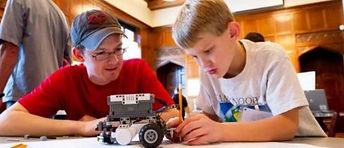How-What-Why Practical Engineering

The How of StatBotics… Solving a Real Problem

When teaching maths, physics, or engineering it is tempting to start with the equation (eg the equation of motion, s=ut+½at²), and then teach the theory. The practical application of the math comes third, if at all. The thing that is missed completely is the description of the original problem that the equation and theory were designed to solve. Following a logical problem solving process, starting with the problem to be solved, is the most natural way for students to learn and, that is the approach that we take in our classes.The What of StatBotics… Exploring the Concepts

There are several subjects in mathematics that are not covered until high school or college, the reason being that the mathematics is too complex for middle school. Specific examples of this are statistics, calculus, and control theory. The mathematics is complex, but the concepts are not. The concepts themselves are well within the grasp of the average middle school student.

Statistics in particular is becoming more and more important in many areas of science, engineering, economics, biology, and commerce. Our robotics class was originally envisioned as a tool for teaching statistics.The Why of Statbotics… Bringing Mathematics into Real Life

Having practical understandings of mathematical concepts will help students put advanced mathematics into context when it is time for them to learn the calculations. We will focus on subjects that are crucial in many areas of science and technology, but that are not typically covered in middle school because of their mathematical complexity. We avoid teaching the complex math, but just teach the concepts using the capabilities and strengths of the EV3 system. At this stage in their learning it is much more important that your child understands the concepts than that they can do the maths. The maths can come later, and will come easier if they learn the concepts now.

6/19-30, 9-6 PM

Week 1

The 16 hours of instruction covering:

• Lego EV3 hardware and software

• Software coding structures and best practices

• Creating your own custom software functions within EV3

• Mathematical modeling of robotic movement

• Linear and non-linear relationships

• Data logging and data analysis

• Comparing motor speed, accuracy, and power

• Descriptive statistics

• The normal distribution and central limit theorem

• Sources of variation

• The Chi Squared test

• Hypothesis testing and statistical confidence

The 24 hours of experimentation with the students designing and building their own robotic machines and putting what they have learned into action. Example machines will be provided to get the students started, including:

• Gearing systems

• Swinging and reciprocating mechanisms

• Cam mechanisms

• Intermittent motion

• Belts and chains

• Wheels and steering

• Walking mechanisms

• Moving through vibration

Week 2

• Characterizing the accuracy of each of the 5 robotics sensors

• Modeling robotic movement using geometry and trigonometry

• Introduction to practical calculus

• How to integrate and differentiate empirically and graphically

• Introduction to control theory

• How to optimize control without introducing instability or oscillation

• The use of calculus within control theory